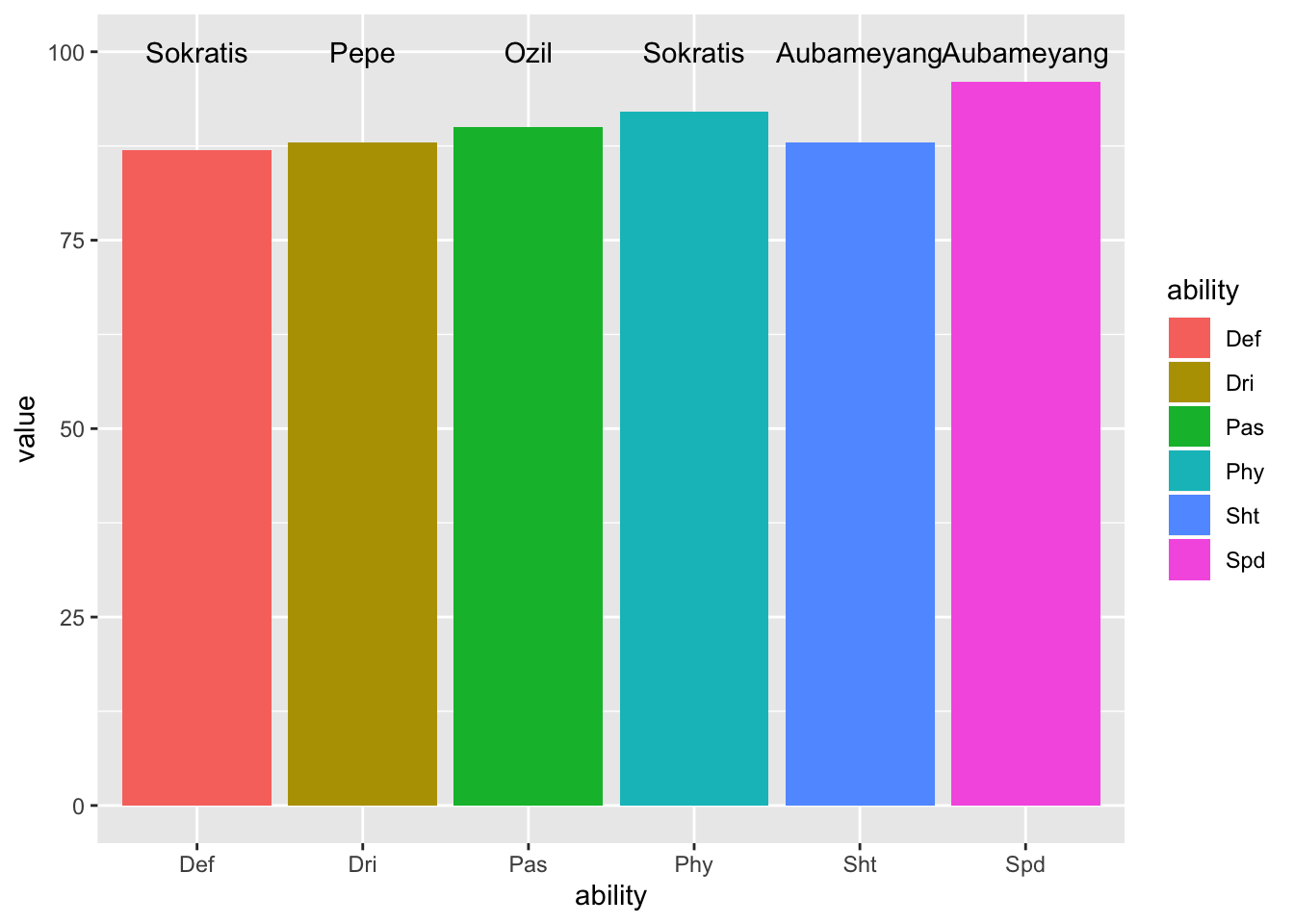# rowwise manipulation of data frame in R

### 2019/09/13

The data frame is used for storing tabular data in R. It is the fundamental data structure in R, especially for tidyverse. Tidy data (data frame), in which each variable in a column and each observation in a row, is used wherever possible througout all the tidyverse packages. Working on column is a very natural and usual operation, and the key ideology in dplyr, a core package of tidyverse. But how to perform rowwise manipulation ? In this post, I will show how to do rowwise operation on data frames using both base R and tidyverse.

## sample data

library(tidyverse)
library(rvest)

url <- "https://www.pesmaster.com/arsenal/pes-2020/team/101/"

html_table() %>%
[[(1) %>%
arrange(desc(Ovr)) %>%
slice(1:10) %>% select(Name, Pas:Dri) %>%
column_to_rownames("Name") %>%
t() %>%
as.data.frame()
names(players) <- stringi::stri_trans_general(names(players), "latin-ascii") %>% # convert to latin-ascii
make.names() %>% # valid names
str_replace_all(".*\\.+", "") # family name

We want to find the the most skillful player of each ability (Pas, Sht, Phy, Def, Spd, and Dri) of ten Arsenal players in PES 2020.

## base R

### apply()

Once rowwise operation is mentioned, the first function comes mind is apply().

index <- apply(players, 1, which.max)
max_ability <- apply(players, 1, max)
skillful_player <- data.frame(
name = names(players)[index],
value = max_ability,
ability = row.names(players)
)

We can use ggplot to visulaize the rusults

player_label <- data.frame(
label = skillful_player$name, x = skillful_player$ability,
y = 100
)
ggplot(skillful_player, aes(x = ability, y = value)) +
geom_col(aes(fill = ability)) +
geom_text(aes(x = x, y = y, label = label), data = player_label)The result shows that the best dribbling player is the new player PEPE, and Ozil is still the best passer, although he have not participated in anyt official match for a long time.

### for loop

Of course someone has to write loops

index_loop <- vector(mode = "double", nrow(players))
for (i in seq_along(index_loop)) {
index_loop[i] <- which.max(players[i, ])
}
max_ability_loop <- vector(mode = "double", nrow(players))
for (i in seq_along(max_ability_loop)) {
max_ability_loop[i] <- max(players[i, ])
}

Apprantely, using for loop is more intuitive, but requires more typing.

### split, then apply and combine

The Next method is split the data frame by row then apply and combine it.

players_split <- split(players, seq_len(nrow(players)))
max_ability <- sapply(players_split, max)
index <- sapply(players_split, which.max)
skillful_player_split <- data.frame(
value = max_ability,
name = names(players)[index],
ability = row.names(players)
)

ggplot(skillful_player_split, aes(x = ability, y = value)) +
geom_col(aes(fill = ability)) +
geom_text(aes(x = x, y = y, label = label), data = player_label)## tidyverse

### pmap() in purrr

purrr::pmap() iterate over multiple arguments simultaneously

which_max <- function(...) {
which.max(c(...))
}
skillful_player_pmap <- players %>%
mutate(index = pmap_int(., which_max),
value = pmap_int(., max),
names = names(players)[index],
ability = row.names(players)
)

ggplot(skillful_player_pmap, aes(x = ability, y = value)) +
geom_col(aes(fill = ability)) +
geom_text(aes(x = x, y = y, label = label), data = player_label)### rowwise() in dplyr

dplyr provides a function rowwise() to preform row-wise operations. However, as mentioned in this issue, we can not use tidyselect operation :, which means that all variables must be explicitly provided for rowwise manipulation. rowwise() is not suitable while there are many varaibles.

skillful_player_rowwise <- players %>%
rowwise() %>%
# mutate(value = max(Aubameyang:Kolasinac)) does not work well
mutate(value = max(Aubameyang, Lacazette, Leno, Sokratis,
Luiz, Ozil, Torreira, Xhaka, Pepe, Kolasinac)
)

Furthermore, we can also transpose the data frame first, and then use apply or dplyr or purrr::map() to perform rowwise operation. Intuitively, this method is more complicated than the method mentioned above, so here we not detail the code.

In summary, purrr::pmap() is using tidyverse and mmore easy to used as part of a pipe. The apply and for loop is more intuitive and efficiency (as shown in here, but requires more typing. dplyr::rowwise() is not suitable while there are many varaibles.